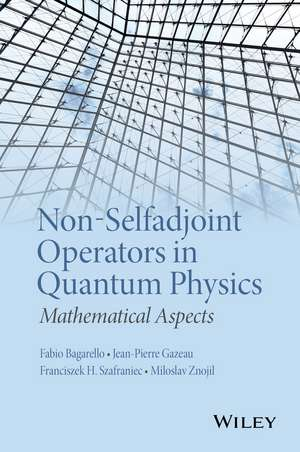# Non-Selfadjoint Operators in Quantum Physics

## matematica si stiinte fizica fizica matematica

5 Review(s)
A unique discussion of mathematical methods with applications to quantum mechanics Non–Selfadjoint Operators in Quantum Physics: Mathematical Aspects presents various mathematical constructions influenced by quantum mechanics and emphasizes the spe...
Cod: 9781118855287 / 314101
Disponibilitate: In stoc
Producator:

## 519.96 RON

A unique discussion of mathematical methods with applications to quantum mechanics Non–Selfadjoint Operators in Quantum Physics: Mathematical Aspects presents various mathematical constructions influenced by quantum mechanics and emphasizes the spectral theory of non–adjoint operators....
Opinia ta despre produs